Courses

# Change Of Phase Class 11 Notes | EduRev

## JEE : Change Of Phase Class 11 Notes | EduRev

The document Change Of Phase Class 11 Notes | EduRev is a part of the JEE Course Physics Class 11.
All you need of JEE at this link: JEE

3. Phase change :

Heat required for the change of phase or state,

Q = mL, L = latent heat.

(a) Latent heat (L) : The heat supplied to a substance which changes its state at constant temperature is called latent heat of the body.

(b) Latent heat of Fusion (Lf) : The heat supplied to a substance which changes it from solid to liquid state at its melting point and 1 atm. pressure is called latent heat of fusion.

(c) Latent heat of vaporisation (Lv) : The heat supplied to a substance which changes it from liquid to vapour state at its boiling point and 1 atm. pressure is called latent heat of vaporization.

If in question latent heat of water are not mentioned and to solve the problem it require to assume that we should consider following values.

Latent heat of ice : L = 80 cal/gm = 80 Kcal/kg = 4200 × 80 J/kg

Latent heat of steam : L = 540 cal/gm = 540 Kcal/kg = 4200 × 540 J/kg

The given figure, represents the change of state by different lines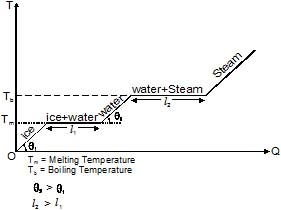Note : If we increases the temperature of liquid (phase)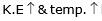­ but at a later time K .E. stop increasing and the phase of the liquid starts changing.

Ex.5 Find amount of heat released if 100 g ice at - 10°C is converted into 120°C, 100 g steam.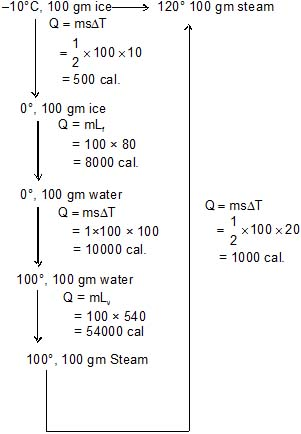Qnet = 73.5 Kcal.

Ex.6 500 gm of water at 80°C is mixed with 100 gm steam at 120°C. Find out the final mixture.

Sol.

120°C steam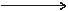100°C steam

Req. heat = 100 ×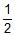×20 = 1 kcal

80°C water100°C water

Req. heat = 500 × 1 × 20 = 10 kcal

100gm steam100 gm water at 100°C

Req. heat = 100 × 540 = 54 kcal

Total heat = 55 kcal.

Remaining heat = 55 - 10 = 45 kcal

Now we have 600 gm water at 100°C ⇒ 4500 = m × 540 ⇒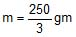So at last we have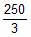gm steam and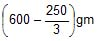of water

Offer running on EduRev: Apply code STAYHOME200 to get INR 200 off on our premium plan EduRev Infinity!

## Physics Class 11

75 videos|222 docs|152 tests

,

,

,

,

,

,

,

,

,

,

,

,

,

,

,

,

,

,

,

,

,

;